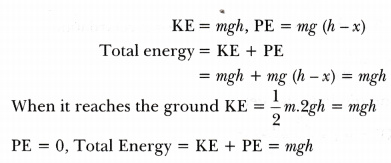# Give one example of each of the following: Small mass but high kinetic energy

1. Give one example of each of the following:
• Small mass but high kinetic energy
• Large mass but low kinetic energy
1. Prove mathematically that the total mechanical energy of a freely falling body in air is conserved.